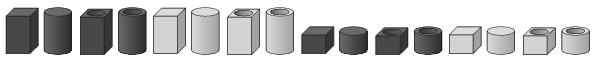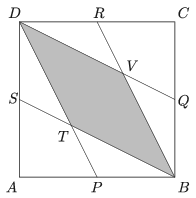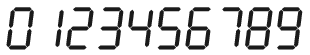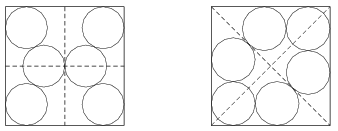Mathematical and Physical Journal
for High Schools
Issued by the MATFUND Foundation
 Already signed up? New to KöMaL?

# KöMaL Problems in Mathematics, October 2018

Show/hide problems of signs:## Problems with sign 'K'

Deadline expired on November 12, 2018.

K. 594. Three two-digit prime numbers are formed by using one of the digits 2, 3, 5, 6, 7 twice and every other digit once. What is the sum of the three numbers?

(6 pont)

solution (in Hungarian), statistics

K. 595. QUARTO is a strategy board game (1991) for two players, invented by the Swiss mathematician Blaiseb Müller. The game includes a set of 16 pieces, each different from all others in some way. The pieces can be divided into two sets of eight by each of four different attributes:

– tall or short;

– black or white;

– round or square;

– hollow or solid at the top.In how many different ways is it possible to select two pieces that agree in exactly two or three attributes?

(6 pont)

solution (in Hungarian), statistics

K. 596. Let $\displaystyle P$, $\displaystyle Q$ and $\displaystyle R$ denote points on sides $\displaystyle AB$, $\displaystyle BC$ and $\displaystyle CA$ of a triangle $\displaystyle ABC$, respectively, such that $\displaystyle AP = AR$, $\displaystyle BP = BQ$ and $\displaystyle CQ = CR$ should hold. How many different sets of such points $\displaystyle P$, $\displaystyle Q$, $\displaystyle R$ may exist for a given triangle $\displaystyle ABC$?

(6 pont)

solution (in Hungarian), statistics

K. 597. The midpoints $\displaystyle P$, $\displaystyle Q$, $\displaystyle R$ and $\displaystyle S$ of the sides of a square $\displaystyle ABCD$ are connected to the vertices as shown in the figure. Determine the ratio of the area of quadrilateral $\displaystyle BVDT$ to that of the square $\displaystyle ABCD$.(6 pont)

solution (in Hungarian), statistics

K. 598. On a digital clock display, digits consist of small illuminated line segments as shown in the figure.The energy consumption of the clock depends on the number of small line segments switched on or off as time elapses. For example, when a 3 changes to a 4, two line segments are switched off and one is switched on, which means 3 switch operations. During a full cycle of $\displaystyle 0, 1, 2, \ldots, 9, 0$, this adds up to a total of 30 switch operations. If the same digit symbols were used to designate the numbers 0 to 9 in some different order, the number of switch operations could be reduced. Find the minimum number of switch operations that could be achieved in a full cycle, and give an example for a possible order of digits.

Proposed by Zs. Ruttkai, the Netherlands

(6 pont)

solution (in Hungarian), statistics## Problems with sign 'C'

Deadline expired on November 12, 2018.

C. 1497. Solve the following simultaneous equations:

\begin{align*} xy & =z,\\ xz & =y,\\ yz & =x. \end{align*}

(5 pont)

solution (in Hungarian), statistics

C. 1498. What is the maximum possible length of the shadow of a 2-metre-tall man on the Earth if the Earth is considered a sphere of radius 6370 km, illuminated by parallel light rays from the Sun?

(5 pont)

solution (in Hungarian), statistics

C. 1499. Find the largest positive integer $\displaystyle n$ for which there exists an appropriate order of the numbers $\displaystyle 1,2,\ldots,n$ such that the large number obtained by writing all the numbers together in a row has the following property: for any pair $\displaystyle a$, $\displaystyle b$ of successive digits, at least one of the two-digit numbers $\displaystyle \overline{ab}$, $\displaystyle \overline{ba}$ is a prime.

(5 pont)

solution (in Hungarian), statistics

C. 1500. On a line segment $\displaystyle AB$, the points $\displaystyle X$ and $\displaystyle Y$ are marked, and the squares $\displaystyle AXPQ$, $\displaystyle XBRS$, $\displaystyle BYWV$ and $\displaystyle YAUT$ are drawn, with their vertices labelled in counterclockwise order. The centres of the squares are denoted by $\displaystyle K$, $\displaystyle L$, $\displaystyle M$ and $\displaystyle N$, respectively. Prove that the line segments $\displaystyle KM$ and $\displaystyle LN$ are perpendicular and equal in length.

German competition problem

(5 pont)

solution (in Hungarian), statistics

C. 1501. Find the longest arithmetic sequence of distinct prime numbers less than 200.

(5 pont)

solution (in Hungarian), statistics

C. 1502. In each figure, there are six circles of equal radius drawn in a unit square. In which arrangement do the circles have a larger radius?German competition problem

(5 pont)

statistics

C. 1503. In a triangle, the squares of the sides $\displaystyle a$, $\displaystyle b$, $\displaystyle c$, in this order, form an arithmetic sequence. Show that the measure of the angle opposite to side $\displaystyle b$ is at most $\displaystyle 60^{\circ}$.

(5 pont)

solution (in Hungarian), statistics## Problems with sign 'B'

Deadline expired on November 12, 2018.

B. 4974. At least how many numbers should be selected out of $\displaystyle 1, 2,\ldots, 10$ so that we can be certain that every such selection will contain a set of numbers whose sum is divisible by 11?

Proposed by S. Róka, Nyíregyháza

(3 pont)

solution (in Hungarian), statistics

B. 4975. Given a point $\displaystyle P$ and four pairwise different lines $\displaystyle e\parallel f$ and $\displaystyle g\parallel h$, construct a line through $\displaystyle P$ that intersects the lines $\displaystyle e$, $\displaystyle f$, $\displaystyle g$, $\displaystyle h$, respectively at points $\displaystyle E$, $\displaystyle F$, $\displaystyle G$, $\displaystyle H$ such that $\displaystyle EF=GH$.

(3 pont)

solution (in Hungarian), statistics

B. 4976. Let $\displaystyle A=\{-4;-3;-2;-1;0;1;2;3;4\}$. First and Second take turns in selecting a number (not selected before) out of the elements of set $\displaystyle A$. The player first collecting three numbers that add up to zero wins the game. Is there a player who has a winning strategy?

Proposed by Á. Bán-Szabó, Budapest

(4 pont)

solution (in Hungarian), statistics

B. 4977. Prove that the orthocentre of the triangle formed by the points of tangency of the incircle on the sides of a right-angled triangle lies on the altitude drawn to the hypotenuse.

(Kvant)

(4 pont)

solution (in Hungarian), statistics

B. 4978. Let $\displaystyle n\ge 3$ be an integer and let $\displaystyle \alpha$ denote an arbitrary real number. Prove that

$\displaystyle \sum_{k=0}^{n-1} \cos^2 \left(\alpha+\frac{2k\pi}{n}\right) = \frac n2.$

(5 pont)

solution (in Hungarian), statistics

B. 4979. In an acute angled triangle $\displaystyle ABC$, $\displaystyle D$ and $\displaystyle E$ are interior points of the sides $\displaystyle AB$ and $\displaystyle AC$, respectively. The line segments $\displaystyle BE$ and $\displaystyle CD$ meet at $\displaystyle F$. Prove that if $\displaystyle BC^2=BD\cdot BA+CE\cdot CA$ then the quadrilateral $\displaystyle ADFE$ is cyclic.

Proposed by S. Róka, Nyíregyháza

(5 pont)

solution, statistics

B. 4980. Let $\displaystyle n>3$ be a positive integer, and let $\displaystyle a_1, a_2, \ldots, a_n$ be positive real numbers. Prove that

$\displaystyle 1 < \frac{a_1}{a_n+a_1+a_2} + \frac{a_2}{a_1+a_2+a_3} + \ldots + \frac{a_n}{a_{n-1}+a_n+a_1} < \left[ \frac{n}{2} \right]$

where the left-hand side of the inequality cannot be replaced by a larger number, and the right-hand side cannot be replaced by a smaller number. ($\displaystyle [x]$ denotes the greatest integer not greater than the number $\displaystyle x$.)

(6 pont)

solution (in Hungarian), statistics

B. 4981. The area of the orthogonal projection of a unit cube onto the plane $\displaystyle xy$ is $\displaystyle A$, and the length of its orthogonal projection onto the $\displaystyle z$-axis is $\displaystyle a$. Prove that $\displaystyle A = a$.

Proposed by P. Erben, Budapest

(6 pont)

solution (in Hungarian), statistics## Problems with sign 'A'

Deadline expired on November 12, 2018.

A. 731. Let $\displaystyle G=(V,E)$ be a tree graph with $\displaystyle n$ vertices, and let $\displaystyle P$ be a set of $\displaystyle n$ points in the plane with no three points collinear. Is it true that for any choice of the graph $\displaystyle G$ and set $\displaystyle P$, we can embed $\displaystyle G$ in $\displaystyle P$, i.e., we can find a bijection $\displaystyle f\colon V\to P$ such that when we draw the line segment $\displaystyle \big[f(x),f(y)\big]$ for all $\displaystyle (x,y)\in E$, no two such segments intersect each other?

Proposed by Benedek Váli, Szeged

(7 pont)

solution, statistics

A. 732. Does there exist an infinite sequence $\displaystyle a_1,a_2,\ldots$ of real numbers which is bounded, not periodic, and satisfies the recursion $\displaystyle a_{n+1}=a_{n-1}a_n+1$?

(7 pont)

statistics

A. 733. Circle $\displaystyle \omega$ lies in the interior of circle $\displaystyle \Omega$, on which a point $\displaystyle X$ moves. The tangents from $\displaystyle X$ to $\displaystyle \omega$ intersect $\displaystyle \Omega$ for the second time at points $\displaystyle A\ne X$ and $\displaystyle B\ne X$. Prove that the lines $\displaystyle AB$ are either all tangent to a fixed circle, or they all pass through a point.

(7 pont)

solution (in Hungarian), statistics